# Vibrationdata Equivalent Static Loads for Random Vibration By

• Slides: 36Vibrationdata Equivalent Static Loads for Random Vibration By Tom Irvine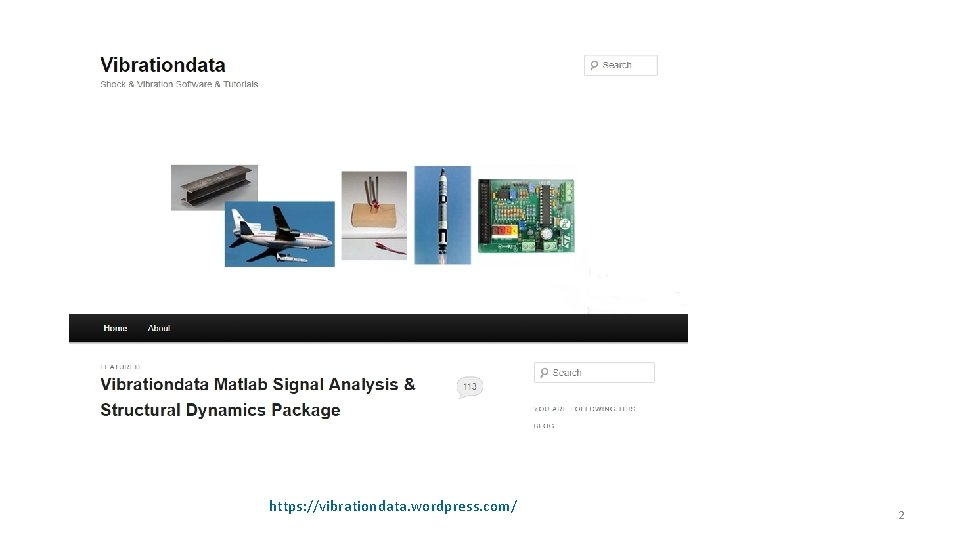https: //vibrationdata. wordpress. com/ 2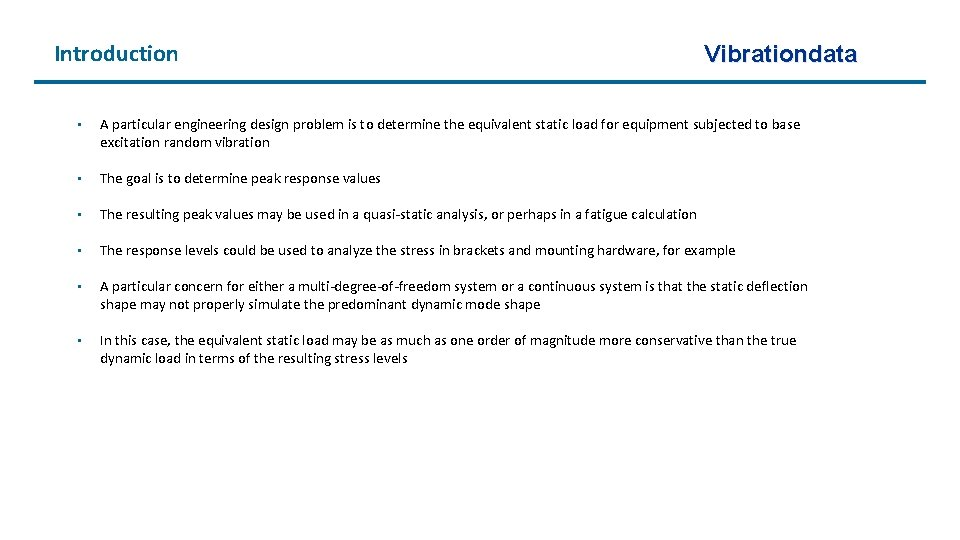Introduction Vibrationdata • A particular engineering design problem is to determine the equivalent static load for equipment subjected to base excitation random vibration • The goal is to determine peak response values • The resulting peak values may be used in a quasi-static analysis, or perhaps in a fatigue calculation • The response levels could be used to analyze the stress in brackets and mounting hardware, for example • A particular concern for either a multi-degree-of-freedom system or a continuous system is that the static deflection shape may not properly simulate the predominant dynamic mode shape • In this case, the equivalent static load may be as much as one order of magnitude more conservative than the true dynamic load in terms of the resulting stress levelsVibrationdata Relevant Parameters • Ideally, the dynamics engineer and the static stress engineer would mutually understand, agree upon, and document the following parameters for the given component: 1. Mass, center-of-gravity, and inertia properties 10. Relative displacement 2. Effective modal mass and participation factors 11. Transmitted force from the base to the component in each of three axes 3. Stiffness 12. Bending moment at the base interface about each of three axes 4. Damping 13. The manner in which the equivalent static loads and moments will be applied to the component, such as point load, body load, distributed load, etc. 5. Natural frequencies 6. Dynamic mode shapes 7. Static deflection shape 8. Response acceleration 14. Dynamic stress and strain at critical locations if the component is best represented as a continuous system 9. Modal velocity 15. Response limit criteria, such as yield stress, ultimate stress, fatigue, or loss of clearanceStress-Velocity Relationship Vibrationdata • Refer to Howard Gaberson’s papers, and Vesta Bateman’s chapter in Harris’ Shock and Vibration Handbook, 6 th edition • Of the three motion parameters (displacement, velocity, and acceleration) describing a shock spectrum, velocity is the parameter of greatest interest from the viewpoint of damage potential • This is because the maximum stresses in a structure subjected to a dynamic load typically are due to the responses of the normal modes of the structure, that is, the responses at natural frequencies • At any given natural frequency, modal stress in a system is proportional to the modal (relative) response velocity = mass density c = speed of sound in the material v = particle velocity at a given point is a constant of proportionality dependent upon the geometry of the structure • Some additional research is needed to further develop this equation so that it can be used for equivalent quasistatic loads for random vibration Bateman, complex equipment or more GabersonStress-Velocity Relationship for Beams Vibrationdata Distance from neutral axis E Elastic modulus A Cross-section Area Mass per volume I Area moment of inertia • Same formula for all common boundary conditions • Maximum stress and maximum velocity may occur at different locations • Assume stress is due to first mode response only • Response may be due to initial conditions, applied force, or base excitation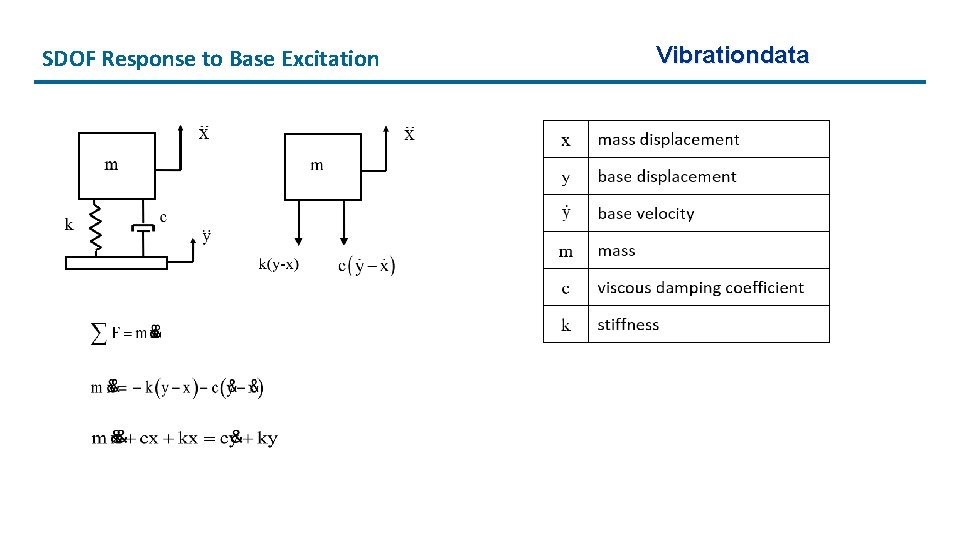SDOF Response to Base Excitation VibrationdataSDOF Response to Base Excitation (cont) • The base velocity and base displacement terms on the righthand side are usually unknown • But the base acceleration is known • Define a relative displacement Vibrationdata z = x - y • Through substitution and algebraic manipulation, the following ordinary differential equation is derived in terms of the relative displacement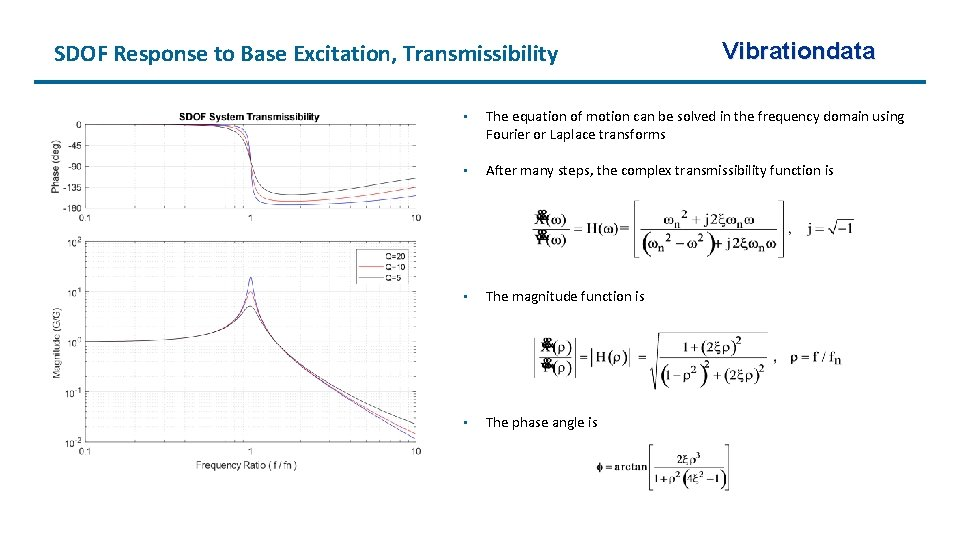SDOF Response to Base Excitation, Transmissibility Vibrationdata • The equation of motion can be solved in the frequency domain using Fourier or Laplace transforms • After many steps, the complex transmissibility function is • The magnitude function is • The phase angle is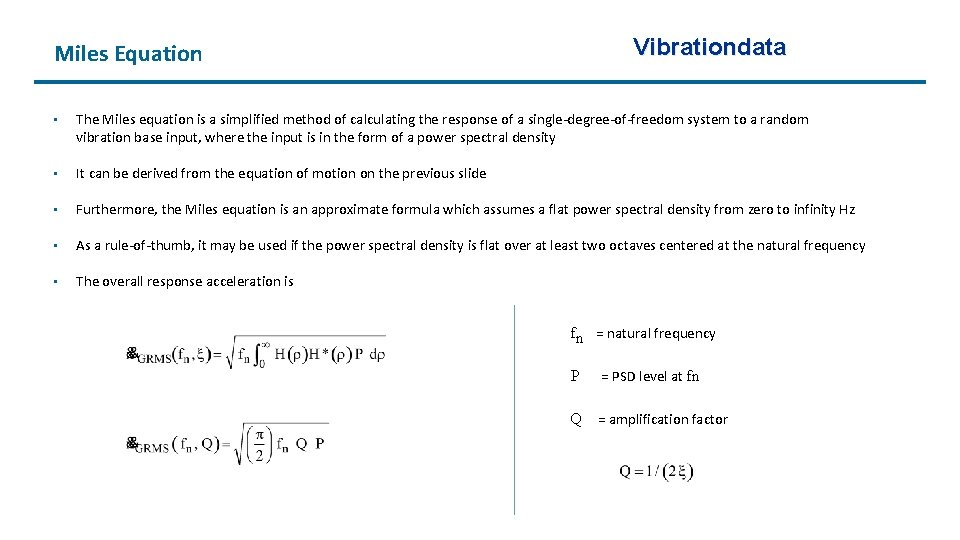Miles Equation Vibrationdata • The Miles equation is a simplified method of calculating the response of a single-degree-of-freedom system to a random vibration base input, where the input is in the form of a power spectral density • It can be derived from the equation of motion on the previous slide • Furthermore, the Miles equation is an approximate formula which assumes a flat power spectral density from zero to infinity Hz • As a rule-of-thumb, it may be used if the power spectral density is flat over at least two octaves centered at the natural frequency • The overall response acceleration is fn = natural frequency P = PSD level at fn Q = amplification factor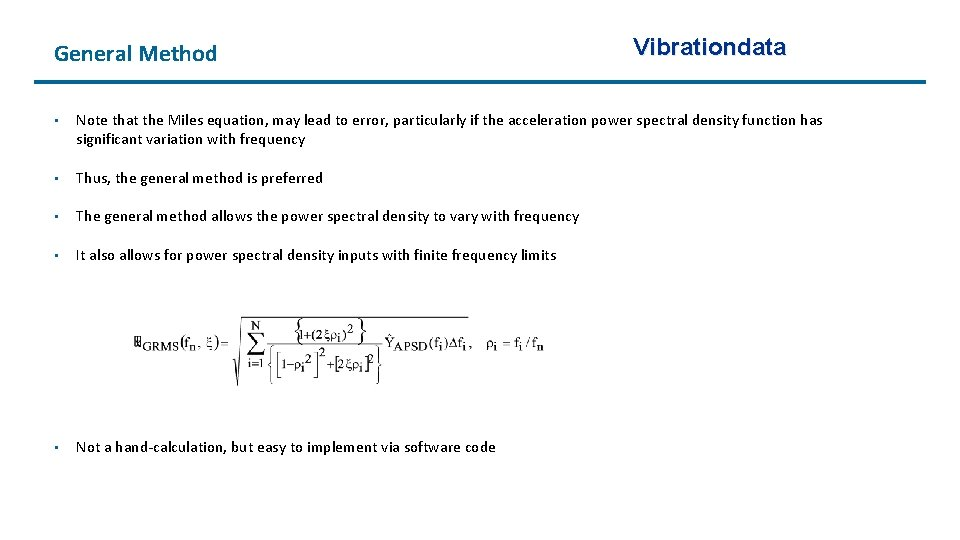General Method Vibrationdata • Note that the Miles equation, may lead to error, particularly if the acceleration power spectral density function has significant variation with frequency • Thus, the general method is preferred • The general method allows the power spectral density to vary with frequency • It also allows for power spectral density inputs with finite frequency limits • Not a hand-calculation, but easy to implement via software code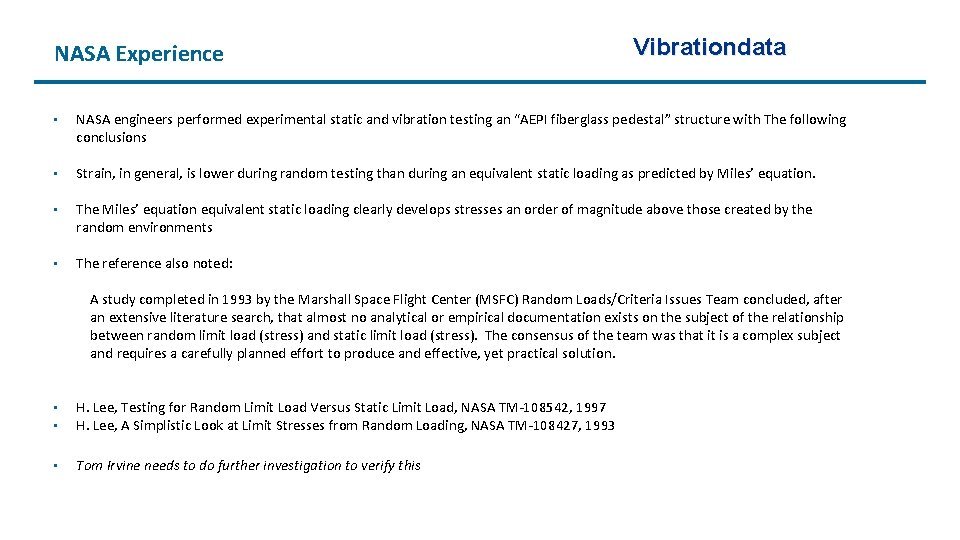Relative Displacement & Spring Force Vibrationdata • Consider a single-degree-of-freedom (SDOF) system subject to a white noise base input and with constant damping • The Miles’ equation set shows the following with respect to the natural frequency fn Response Acceleration ∝ f n 0. 5 Relative Displacement ∝ 1/ f n 1. 5 Relative Displacement = Response Acceleration / ωn 2 • Consider that the stress is proportional to the force transmitted through the mounting spring • The spring force F is equal to the stiffness k times the relative displacement z F = k z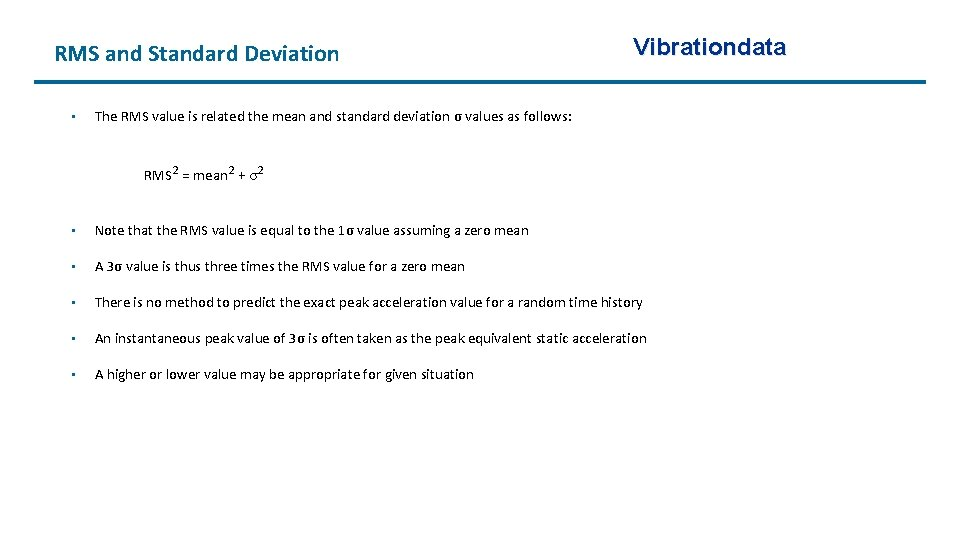RMS and Standard Deviation • Vibrationdata The RMS value is related the mean and standard deviation σ values as follows: RMS 2 = mean 2 + σ2 • Note that the RMS value is equal to the 1σ value assuming a zero mean • A 3σ value is thus three times the RMS value for a zero mean • There is no method to predict the exact peak acceleration value for a random time history • An instantaneous peak value of 3σ is often taken as the peak equivalent static acceleration • A higher or lower value may be appropriate for given situationSample Design Guidelines for Peak Response Acceleration or Transmitted Force Reference Design Level Vibrationdata Comment Himelblau, et al 3 However, the response may be non-linear and non-Gaussian Fackler 3 3 is the usual assumption for the equivalent peak sinusoidal level Luhrs 3 Theoretically, any large acceleration may occur NASA 3 for STS Payloads 2 for ELV Payloads Minimum Probability Level Requirements Mc. Donnell Douglas 4 Equivalent Static Load Scharton & Pankow 5 Upcoming slide Di. Maggio, Sako, Rubin N Upcoming slide Ahlin Cn Upcoming slide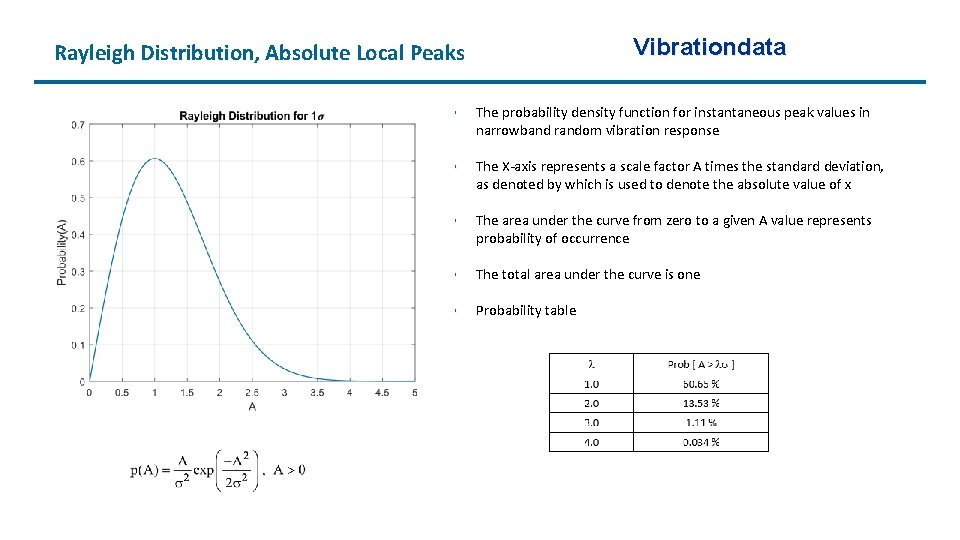Vibrationdata Rayleigh Distribution, Absolute Local Peaks • The probability density function for instantaneous peak values in narrowband random vibration response • The X-axis represents a scale factor A times the standard deviation, as denoted by which is used to denote the absolute value of x • The area under the curve from zero to a given A value represents probability of occurrence • The total area under the curve is one • Probability table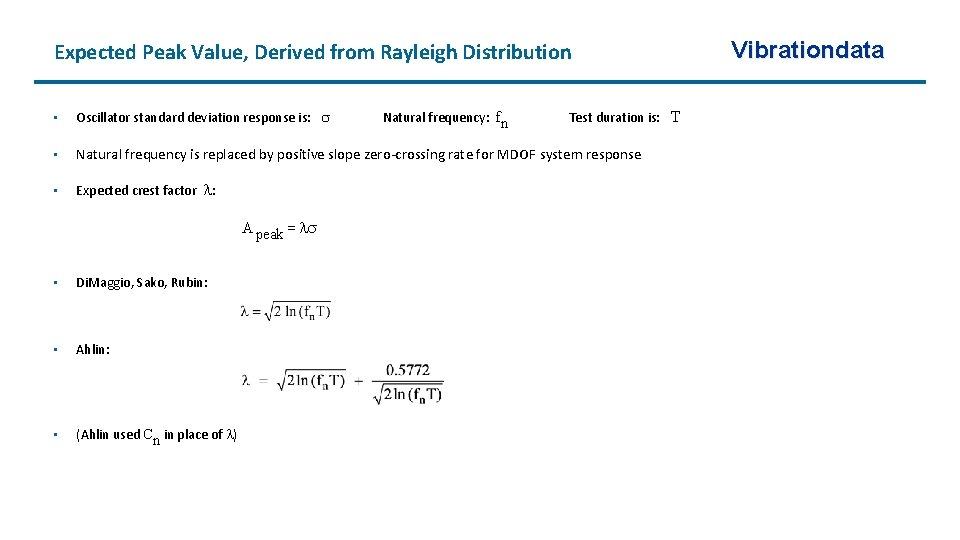Expected Peak Value, Derived from Rayleigh Distribution • Oscillator standard deviation response is: Natural frequency: fn Test duration is: T • Natural frequency is replaced by positive slope zero-crossing rate for MDOF system response • Expected crest factor : A peak = • Di. Maggio, Sako, Rubin: • Ahlin: • (Ahlin used Cn in place of ) Vibrationdata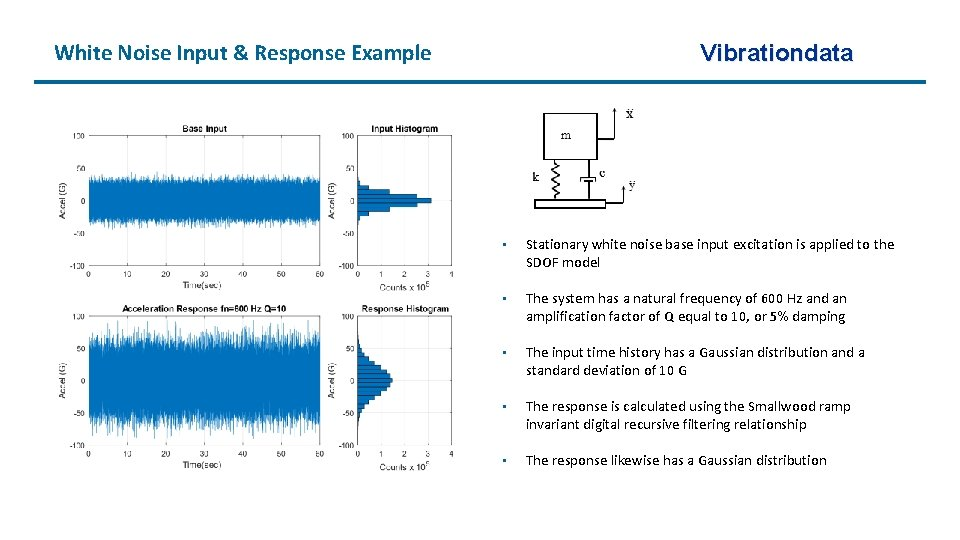White Noise Input & Response Example Vibrationdata • Stationary white noise base input excitation is applied to the SDOF model • The system has a natural frequency of 600 Hz and an amplification factor of Q equal to 10, or 5% damping • The input time history has a Gaussian distribution and a standard deviation of 10 G • The response is calculated using the Smallwood ramp invariant digital recursive filtering relationship • The response likewise has a Gaussian distributionRayleigh Distribution of Local Response Peaks Vibrationdata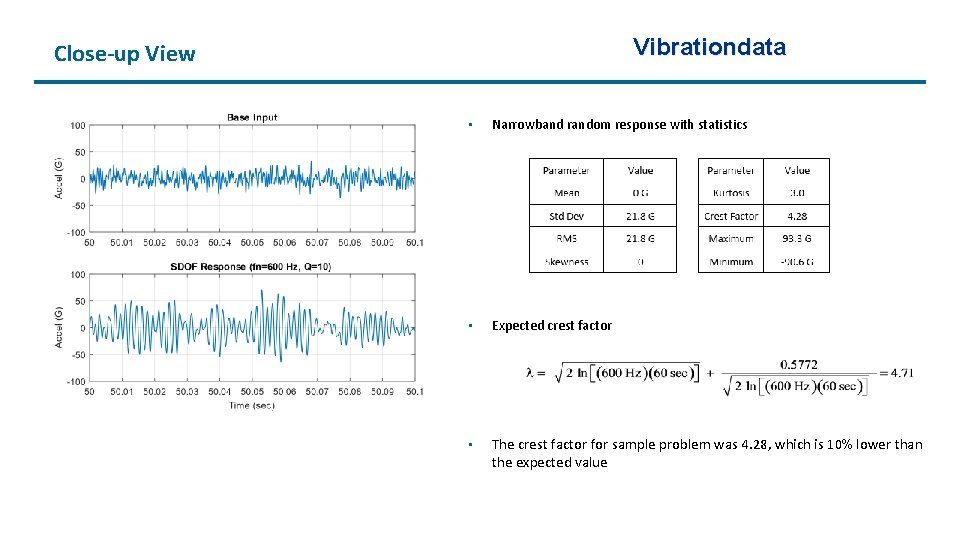Vibrationdata Close-up View • Narrowband random response with statistics • Expected crest factor • The crest factor for sample problem was 4. 28, which is 10% lower than the expected valueVibrationdata Crest Factor Numerical Study • The white noise synthesis and response calculation steps for the previous example were repeated 20, 000 times • A crest factor was calculated for each trial • The crest factor histogram has a shape somewhat similar to a Rayleigh distribution, but further investigation is needed to properly characterize it • The crest factors ranged from 4. 14 to 6. 62, with a mean of 4. 76 • This experimental mean value was 1. 1% higher than theoretical 4. 71 value • Stationary random vibration time history with Gaussian distributions typically have peaks ranging from 4σ to 5σ, but higher crest values may also occur • This is true both for broadband inputs and narrowband responses • Designing structures and components for 3σ vibration loads is thus insufficient, even though this is a common rule-of-thumb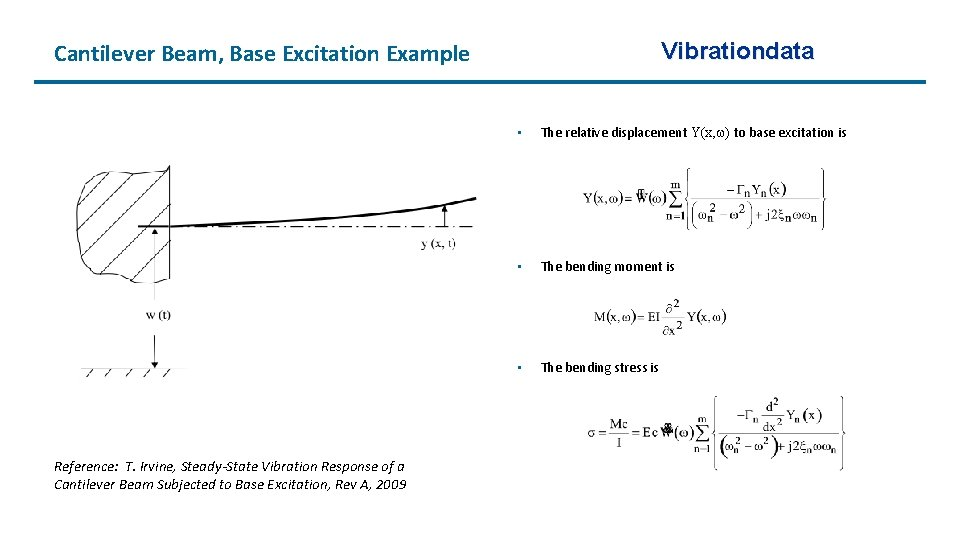Vibrationdata Cantilever Beam, Base Excitation Example Reference: T. Irvine, Steady-State Vibration Response of a Cantilever Beam Subjected to Base Excitation, Rev A, 2009 • The relative displacement Y(x, ) to base excitation is • The bending moment is • The bending stress is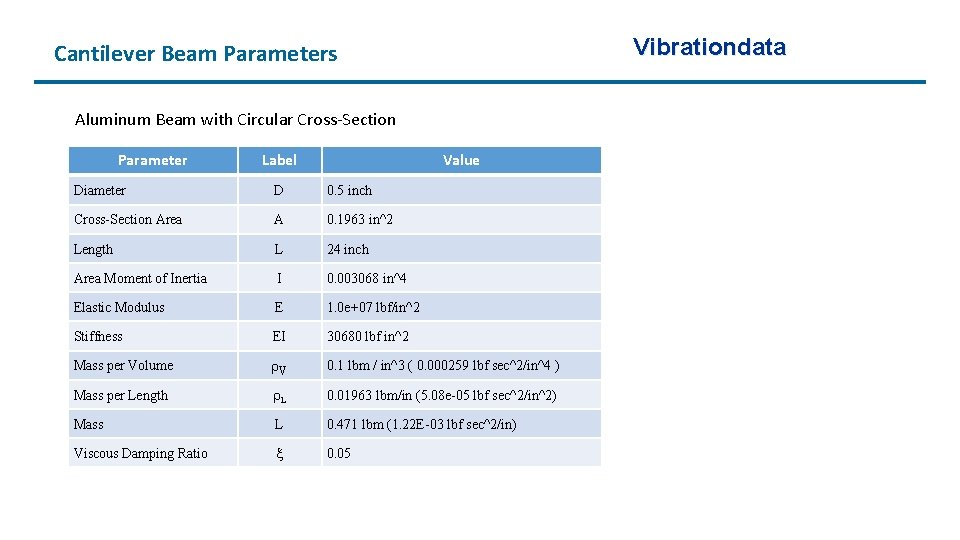Vibrationdata Cantilever Beam Parameters Aluminum Beam with Circular Cross-Section Parameter Label Value Diameter D 0. 5 inch Cross-Section Area A 0. 1963 in^2 Length L 24 inch Area Moment of Inertia I 0. 003068 in^4 Elastic Modulus E 1. 0 e+07 lbf/in^2 Stiffness EI 30680 lbf in^2 Mass per Volume V 0. 1 lbm / in^3 ( 0. 000259 lbf sec^2/in^4 ) Mass per Length L 0. 01963 lbm/in (5. 08 e-05 lbf sec^2/in^2) Mass L 0. 471 lbm (1. 22 E-03 lbf sec^2/in) Viscous Damping Ratio 0. 05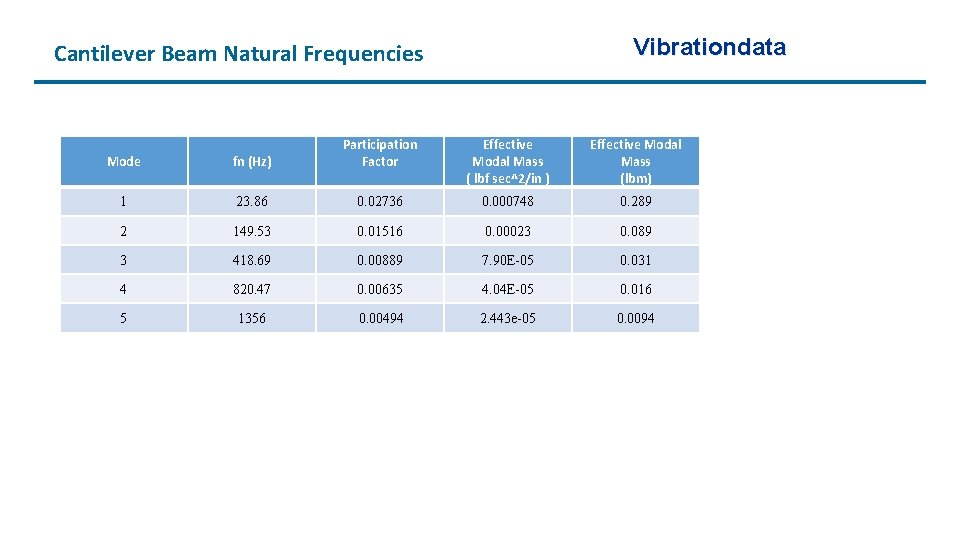Vibrationdata Cantilever Beam Natural Frequencies Mode fn (Hz) Participation Factor Effective Modal Mass ( lbf sec^2/in ) Effective Modal Mass (lbm) 1 23. 86 0. 02736 0. 000748 0. 289 2 149. 53 0. 01516 0. 00023 0. 089 3 418. 69 0. 00889 7. 90 E-05 0. 031 4 820. 47 0. 00635 4. 04 E-05 0. 016 5 1356 0. 00494 2. 443 e-05 0. 0094Acceleration Transmissibility VibrationdataVelocity & Bending Stress Transmissibility VibrationdataBase Input PSD to Cantilever Beam & Accel VRS Vibrationdata Base Input PSD 14. 1 GRMS Overall 180 seconds VRS is for an Single-degree-of-freedom (SDOF) System Freq (Hz) Accel (G^2/Hz) 10 0. 1 2000 0. 1PV & Relative Displacement VRS Vibrationdata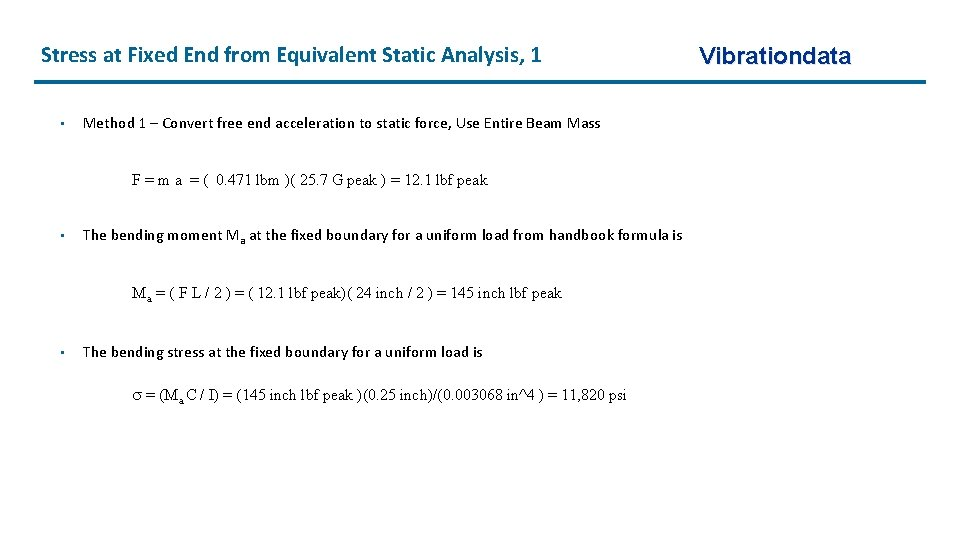Stress at Fixed End from Equivalent Static Analysis, 1 • Method 1 – Convert free end acceleration to static force, Use Entire Beam Mass F = m a = ( 0. 471 lbm )( 25. 7 G peak ) = 12. 1 lbf peak • The bending moment Ma at the fixed boundary for a uniform load from handbook formula is Ma = ( F L / 2 ) = ( 12. 1 lbf peak)( 24 inch / 2 ) = 145 inch lbf peak • The bending stress at the fixed boundary for a uniform load is = (Ma C / I) = (145 inch lbf peak )(0. 25 inch)/(0. 003068 in^4 ) = 11, 820 psi Vibrationdata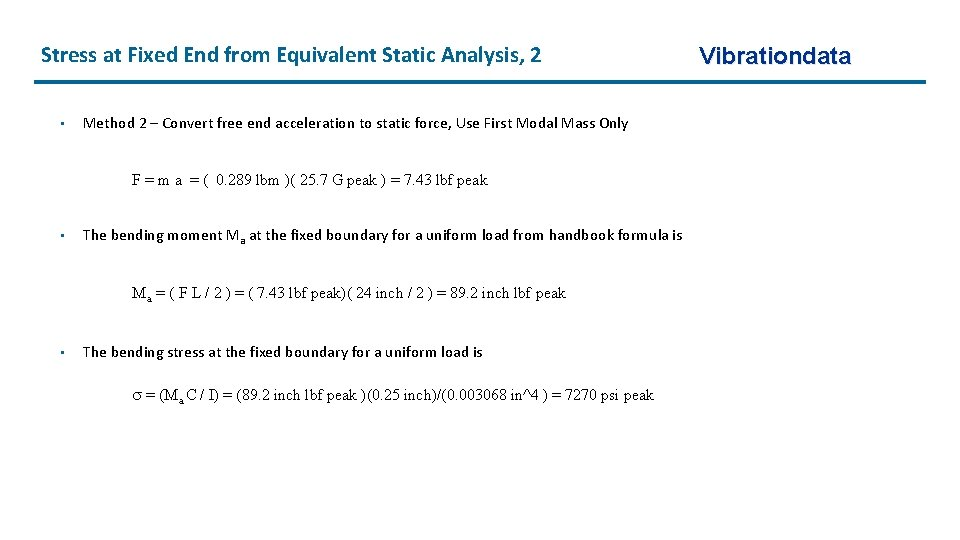Stress at Fixed End from Equivalent Static Analysis, 2 • Method 2 – Convert free end acceleration to static force, Use First Modal Mass Only F = m a = ( 0. 289 lbm )( 25. 7 G peak ) = 7. 43 lbf peak • The bending moment Ma at the fixed boundary for a uniform load from handbook formula is Ma = ( F L / 2 ) = ( 7. 43 lbf peak)( 24 inch / 2 ) = 89. 2 inch lbf peak • The bending stress at the fixed boundary for a uniform load is = (Ma C / I) = (89. 2 inch lbf peak )(0. 25 inch)/(0. 003068 in^4 ) = 7270 psi peak Vibrationdata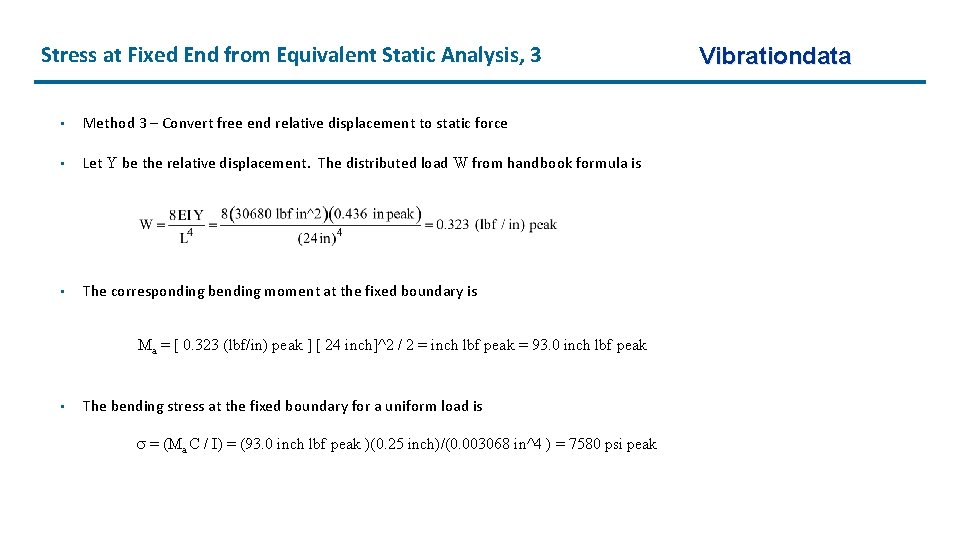Stress at Fixed End from Equivalent Static Analysis, 3 • Method 3 – Convert free end relative displacement to static force • Let Y be the relative displacement. The distributed load W from handbook formula is • The corresponding bending moment at the fixed boundary is Ma = [ 0. 323 (lbf/in) peak ] [ 24 inch]^2 / 2 = inch lbf peak = 93. 0 inch lbf peak • The bending stress at the fixed boundary for a uniform load is = (Ma C / I) = (93. 0 inch lbf peak )(0. 25 inch)/(0. 003068 in^4 ) = 7580 psi peak VibrationdataStress at Fixed End from Stress Velocity, 4 • Stress-Velocity relationship for beam Vibrationdata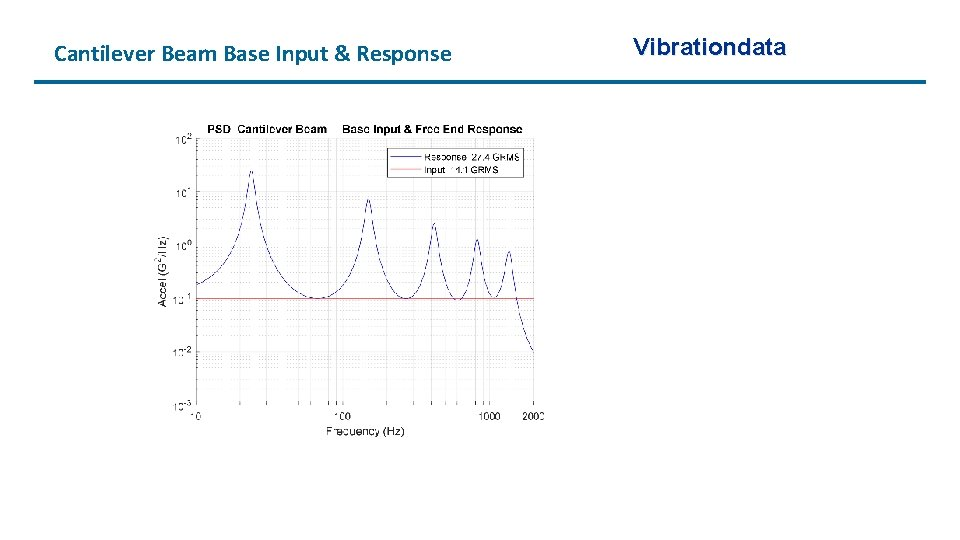Cantilever Beam Base Input & Response VibrationdataCantilever Beam Stress Response at Fixed-End Continuous Model, One Mode Solution Vibrationdata Continuous Model, Five Mode SolutionContinuous Model, Expected Peak Stress • The expected crest factor is • Expected peak stress from continuous beam mode with one mode is (1977 psi RMS )(4. 23) = 8366 psi peak VibrationdataVibrationdata Peak Stress Comparison • The continuous beam value is considered as the “exact” value • Methods 1 through 4 each used a SDOF model approach from vibration response spectrum • Method 1 over-predicted the stress • Methods 2 through 4 under-predicted the stress • Fundamental bending mode shape is not the same as static deflection shape • Cantilever beam is not a spring-mass SDOF system Method Stress (psi peak) Method 1, VRS Acceleration 11820 Method 2, VRS Acceleration 7270 Method 3, VRS Relative Displacement 7580 Method 4, VRS Pseudo Velocity 6686 Method 5, Continuous Beam 8366